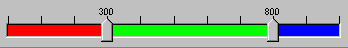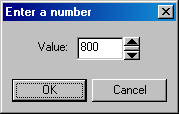﻿ Multi-slider Control Dialog | Appendix | Common Dialog C++ Class Library Help
In This Topic ▼

# Multi-slider Control Dialog

Use the Multi-slider control to set range values for a number of dialogs.Use the Multi-slider control to specify the following:

## Three values where the:

• First value will be the result of subtracting the first marker value from the Multi-slider start value.
• Second value will be the result of subtracting the second marker value from the first marker value.
• Third value will be the result of subtracting the second marker value from the Multi-slider end value.

## The Range within two values where the:

• Range beginning value will be the first marker value.
• Range end value will be the second marker value.

## How to use

You can change the values by changing the markers' positions.

Changing the markers' positions can be done by dragging the marker to a new position. You can also right-click on the marker you want to change and display the Value dialog, which lets you set the new marker value (position).Help Version 21.0.2021.7.2